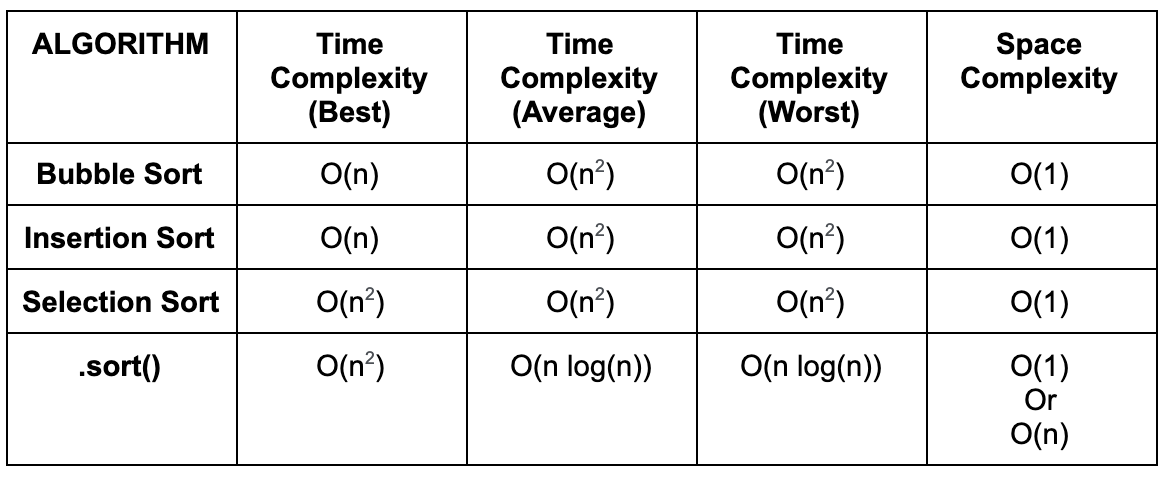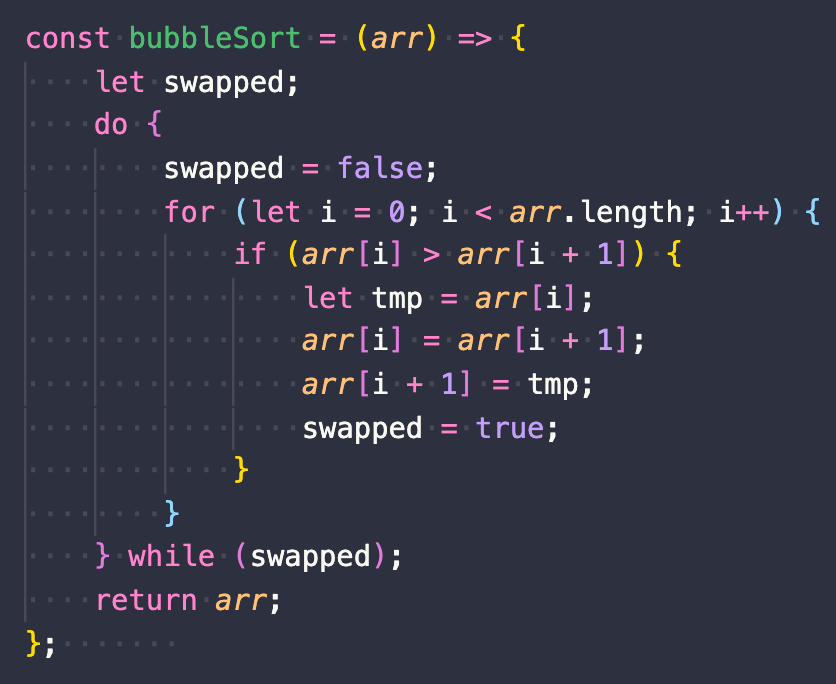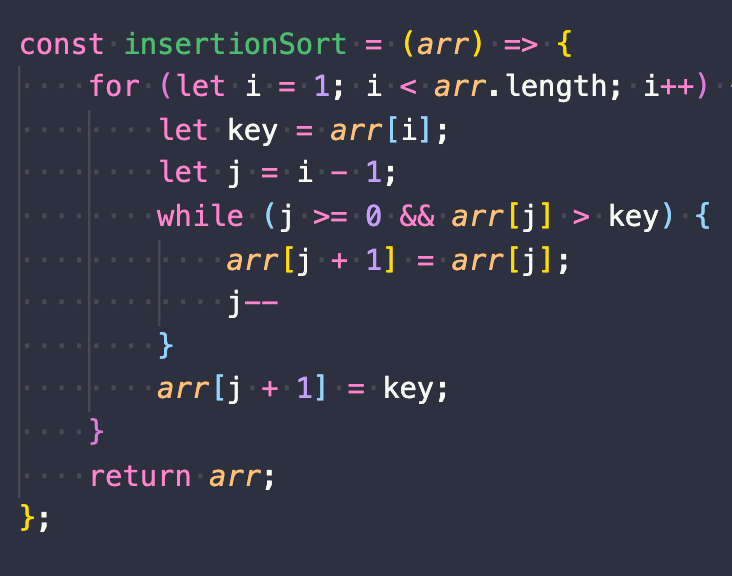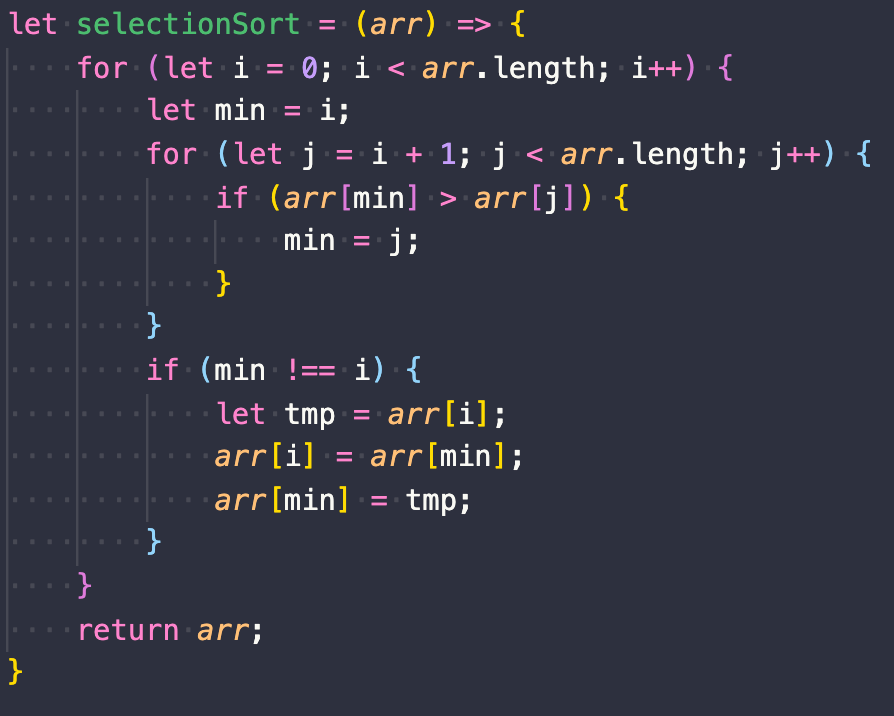# Sorting Algorithms: Buble, Insertion, and Selection Sort(Part 1)

Although JavaScript has its own built in .sort() function, there are different situations where using a sorting algorithm may be useful. In this article we will go into depth about Insertion Sort, Buble Sort, and Selection Sort..sort() may also act differently depending on web browser
• Bubble Sort: step through list comparing adjacent elements, and swapping them is they are in the wrong order, good if data is already partially sorted
• Insertion Sort: inserts numbers from the unsorted list to the sorted list one at a time, good for online data (live data)
• Selection Sort: iterating through the array, finding the smallest value and swapping it with the first unsorted number in the list, not efficient, but it is simple

Bubble Sort: we repeatedly step through the list, comparing adjacent elements and swapping them if they are in the wrong order. In this example we would swap 15 with 3, then 15 with 2, and continue until 15 is at the end of the list. We will continue to do the same with all values until we have an ordered list. Bubble sort has an average time complexity of O(n²).We start our bubble sort by iterating through the array, if the first number in our list is greater than the following number we swap the two (arr[i] = arr[i+1], arr[i+1]=tmp). If the first number is the largest number in the list, we will iterate through the entire for loop moving that number to the end. If not we move on to the next number and do the same until the entire list is sorted (no swaps occur and swapped=false).

Insertion Sort: creates a sorted list by inserting numbers one at a time from the original list. Insertion sort runs in O(n²), or quadratic time (in the worst case).

First we separate our list into sorted, and non sorted. Index 0 will initially be the only sorted value, all following indices will be part of the unsorted list.

We then will check the first value (key) in the non sorted list, and insert it in the sorted list, by swapping it to the left of any larger values.

Step by step:

• 3 is less than 15, so we move 3 to the left of 15
• Now that 3 is in the sorted list we move on to 2. 2 is less than 15, so we move 2 to the left of 15. 2 is less than 3, so we move it to the left of 3.
• Now that 2 is in the sorted list we move on to 9. 9 is less than 15, so we move it to the left. 9 is greater than 3, so it stays.
• Once 9 is in the sorted list we continue to follow the same procedure until we get a folly sorted list as you can see to the below.Here the key is the first number in the unsorted list. The while loop will run until it hits a number to the left with a lesser value than that of the key, or when j becomes negative. In the while loop we swap the value of the key with the value of the preceding number, and iterating to the left (j — )continuing to move the larger values to the right(arr[j+1]=arr[j]). Once we hit the end of the array (j≥0) or the number to the left of the key is smaller than the key (arr[j]>key), the value of the key is inserted to the correct place in the sorted array(arr[j+1]=key).

Selection Sort: iterating through the array, finding the smallest value (that has not been sorted) and swapping it with the first unsorted number in the array. Selection sort has a O(n²) time complexity.

Overview: here we start with 15, when iterating through the array 2 is the smallest number. For that reason 2 and 15 are swapped. Then we move on to 3, there are no smaller numbers in the array so 3 does not move. Then we move on to 15, 5 is the smallest number in the unsorted portion of the array, so we swap the two. We continue to do this until all values are sorted.Here we iterate though the array(first for loop), and for each element we check through the unsorted array (second for loop). We compare the first unsorted number (arr[min] aka arr[i],where i=0)to the number on the right(arr[min]>arr[j]) if it is greater we save the number to the right as the smallest (min=j). Once we found the smallest number to the right we swap the smaller number with the first unsorted number (arr[i]=arr[min], arr[min]=tmp). This is done for every number until the list is sorted, starting the comparison one index forward each time.

--

--